Anzeige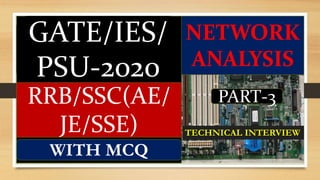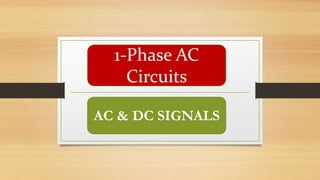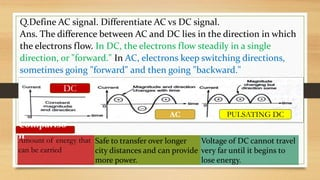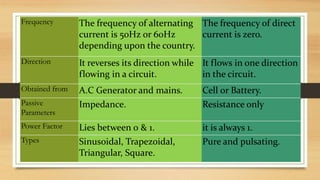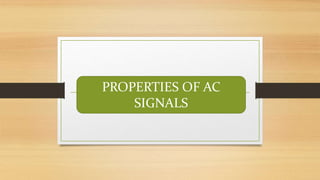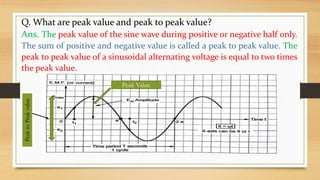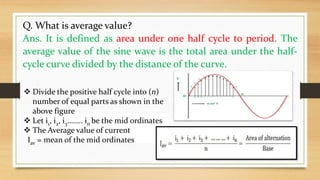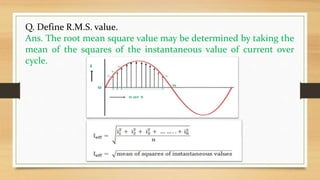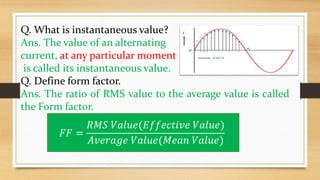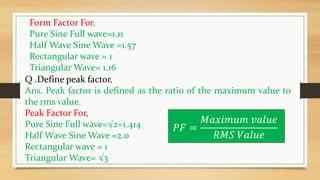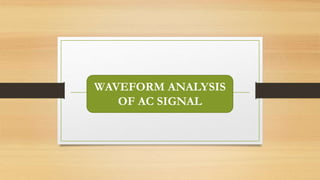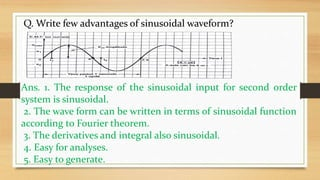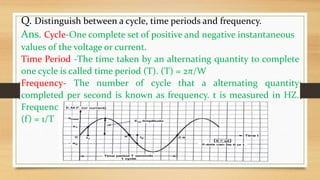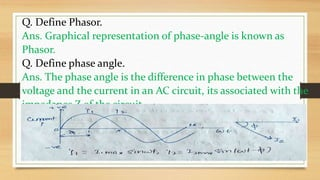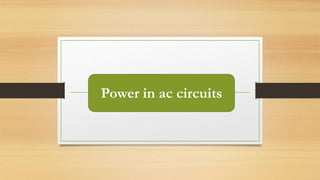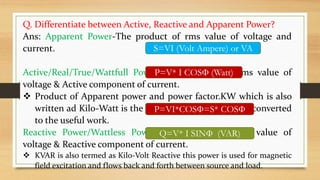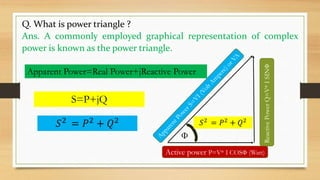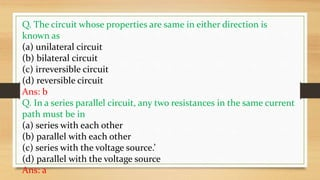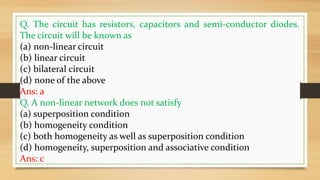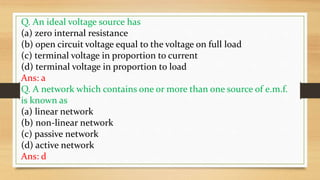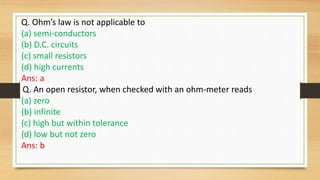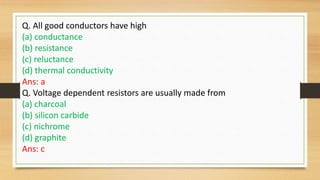1 von 23
Anzeige

### NETWORK ANALYSIS PART 3 For GATE IES PSU -2020 RRB/SSC AE JE TECHNICAL INTERVIEW

1. GATE/IES/ PSU-2020 RRB/SSC(AE/ JE/SSE) WITH MCQ NETWORK ANALYSIS PART-3 TECHNICAL INTERVIEW
2. 1-Phase AC Circuits AC & DC SIGNALS
3. Q.Define AC signal. Differentiate AC vs DC signal. Ans. The difference between AC and DC lies in the direction in which the electrons flow. In DC, the electrons flow steadily in a single direction, or "forward." In AC, electrons keep switching directions, sometimes going "forward" and then going "backward." Amount of energy that can be carried Safe to transfer over longer city distances and can provide more power. Voltage of DC cannot travel very far until it begins to lose energy. Compariso n DC AC PULSATING DC
4. Frequency The frequency of alternating current is 50Hz or 60Hz depending upon the country. The frequency of direct current is zero. Direction It reverses its direction while flowing in a circuit. It flows in one direction in the circuit. Obtained from A.C Generator and mains. Cell or Battery. Passive Parameters Impedance. Resistance only Power Factor Lies between 0 & 1. it is always 1. Types Sinusoidal, Trapezoidal, Triangular, Square. Pure and pulsating.
5. PROPERTIES OF AC SIGNALS
6. Q. What are peak value and peak to peak value? Ans. The peak value of the sine wave during positive or negative half only. The sum of positive and negative value is called a peak to peak value. The peak to peak value of a sinusoidal alternating voltage is equal to two times the peak value. PeaktoPeakvalue Peak Value
7. Q. What is average value? Ans. It is defined as area under one half cycle to period. The average value of the sine wave is the total area under the half- cycle curve divided by the distance of the curve.  Divide the positive half cycle into (n) number of equal parts as shown in the above figure  Let i1, i2, i3…….. in be the mid ordinates  The Average value of current Iav = mean of the mid ordinates
8. Q. Define R.M.S. value. Ans. The root mean square value may be determined by taking the mean of the squares of the instantaneous value of current over cycle.
9. Q. What is instantaneous value? Ans. The value of an alternating current, at any particular moment is called its instantaneous value. Q. Define form factor. Ans. The ratio of RMS value to the average value is called the Form factor. 𝐹𝐹 = 𝑅𝑀𝑆 𝑉𝑎𝑙𝑢𝑒(𝐸𝑓𝑓𝑒𝑐𝑡𝑖𝑣𝑒 𝑉𝑎𝑙𝑢𝑒) 𝐴𝑣𝑒𝑟𝑎𝑔𝑒 𝑉𝑎𝑙𝑢𝑒(𝑀𝑒𝑎𝑛 𝑉𝑎𝑙𝑢𝑒)
10. Q .Define peak factor. Ans. Peak factor is defined as the ratio of the maximum value to the rms value. Peak Factor For, Pure Sine Full wave=√2=1.414 Half Wave Sine Wave =2.0 Rectangular wave = 1 Triangular Wave= √3 𝑃𝐹 = 𝑀𝑎𝑥𝑖𝑚𝑢𝑚 𝑣𝑎𝑙𝑢𝑒 𝑅𝑀𝑆 𝑉𝑎𝑙𝑢𝑒 Form Factor For, Pure Sine Full wave=1.11 Half Wave Sine Wave =1.57 Rectangular wave = 1 Triangular Wave= 1.16
11. WAVEFORM ANALYSIS OF AC SIGNAL
12. Q. Write few advantages of sinusoidal waveform? Ans. 1. The response of the sinusoidal input for second order system is sinusoidal. 2. The wave form can be written in terms of sinusoidal function according to Fourier theorem. 3. The derivatives and integral also sinusoidal. 4. Easy for analyses. 5. Easy to generate.
13. Q. Distinguish between a cycle, time periods and frequency. Ans. Cycle-One complete set of positive and negative instantaneous values of the voltage or current. Time Period -The time taken by an alternating quantity to complete one cycle is called time period (T). (T) = 2π/W Frequency- The number of cycle that a alternating quantity completed per second is known as frequency. t is measured in HZ. Frequency (f) = 1/T
14. Q. Define Phasor. Ans. Graphical representation of phase-angle is known as Phasor. Q. Define phase angle. Ans. The phase angle is the difference in phase between the voltage and the current in an AC circuit, its associated with the impedance Z of the circuit.
15. Power in ac circuits
16. Q. Differentiate between Active, Reactive and Apparent Power? Ans: Apparent Power-The product of rms value of voltage and current. Active/Real/True/Wattfull Power= The product of rms value of voltage & Active component of current.  Product of Apparent power and power factor.KW which is also written ad Kilo-Watt is the real power that is actually converted to the useful work. Reactive Power/Wattless Power=The product of rms value of voltage & Reactive component of current.  KVAR is also termed as Kilo-Volt Reactive this power is used for magnetic field excitation and flows back and forth between source and load. S=VI (Volt Ampere) or VA P=V* I COSФ (Watt) P=VI*COSФ=S* COSФ Q=V* I SINФ (VAR)
17. Active power P=V* I COSФ (Watt) ReactivePowerQ=V*ISINФ Ф Apparent Power=Real Power+jReactive Power S=P+jQ 𝑆2 = 𝑃2 + 𝑄2 Q. What is power triangle ? Ans. A commonly employed graphical representation of complex power is known as the power triangle. 𝑆2 = 𝑃2 + 𝑄2
18. MCQ FOR PRACTICES
19. Q. The circuit whose properties are same in either direction is known as (a) unilateral circuit (b) bilateral circuit (c) irreversible circuit (d) reversible circuit Ans: b Q. In a series parallel circuit, any two resistances in the same current path must be in (a) series with each other (b) parallel with each other (c) series with the voltage source.’ (d) parallel with the voltage source Ans: a
20. Q. The circuit has resistors, capacitors and semi-conductor diodes. The circuit will be known as (a) non-linear circuit (b) linear circuit (c) bilateral circuit (d) none of the above Ans: a Q. A non-linear network does not satisfy (a) superposition condition (b) homogeneity condition (c) both homogeneity as well as superposition condition (d) homogeneity, superposition and associative condition Ans: c
21. Q. An ideal voltage source has (a) zero internal resistance (b) open circuit voltage equal to the voltage on full load (c) terminal voltage in proportion to current (d) terminal voltage in proportion to load Ans: a Q. A network which contains one or more than one source of e.m.f. is known as (a) linear network (b) non-linear network (c) passive network (d) active network Ans: d
22. Q. Ohm’s law is not applicable to (a) semi-conductors (b) D.C. circuits (c) small resistors (d) high currents Ans: a Q. An open resistor, when checked with an ohm-meter reads (a) zero (b) infinite (c) high but within tolerance (d) low but not zero Ans: b
23. Q. All good conductors have high (a) conductance (b) resistance (c) reluctance (d) thermal conductivity Ans: a Q. Voltage dependent resistors are usually made from (a) charcoal (b) silicon carbide (c) nichrome (d) graphite Ans: c
Anzeige